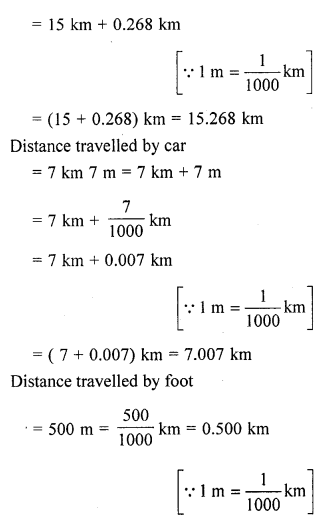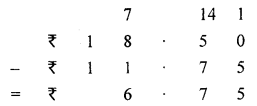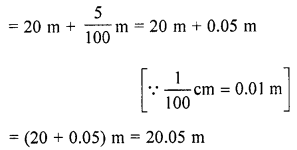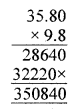## ML Aggarwal Class 6 Solutions for ICSE Maths Chapter 7 Decimals Ex 7.5

Question 1.
Express as rupees using decimals:
(i) 75 paise
(ii) 1025 paise
(ii) 63 rupees 9 paise
Solution:
(i) 75 paise = ₹$$\frac{75}{100}$$ = ₹0.75
(ii) 1025 paise = ₹$$\frac{1025}{100}$$ = ₹10.25
(iii) 63 rupees 9 paise = ₹$$\frac{6309}{100}$$ = ₹63.09

Question 2.
Express as cm using decimals:
(i) 8 mm
(ii) 263 mm
(iii) 13 cm 3 mm
Solution:
(i) 8 mm = $$\frac{8}{10}$$ cm = 0.8 cm
(ii) 263 mm = $$\frac{263}{10}$$cm = 26.3 cm
(iii) 13 cm 3 mm = 13 cm × $$\frac{3}{10}$$ cm = 13.3 cm

Question 3.
Express as metres using decimals:
(i) 6 cm
(ii) 528 cm
(iii) 7 m 55 cm
Solution:
(i) 6 cm = $$\frac{6}{100}$$ m = 0.06 m
(ii) 528 cm = $$\frac{528}{100}$$ m = 5.28 m
(iii) 7 m 55 cm = 7 m + 55 cm
= 7 m + $$\frac{55}{100}$$m = (7 + .55) m = 7.55 m

Question 4.
Express as km using decimals:
(i) 5 m
(ii) 888 m
(iii) 15 km 88 m
Solution:
(i) 5 m = $$\frac{5}{1000}$$ km = 0.005 km
(ii) 888 m = $$\frac{888}{1000}$$km = 0.888 km
(iii) 15 km 88 m = 15 km + 88 m
= 15 km + $$\frac{88}{1000}$$ km = (15 + 0.88) kmsss
= 15.088 km

Question 5.
Express as kg using decimals:
(i) 37 g
(ii) 100 g
(iii) 5 kg 8 g
Solution:
(i) 37g = $$\frac{37}{1000}$$ kg = 0.037 kg
(ii) 100 g = $$\frac{100}{1000}$$kg = 0.1 kg
(iii) 5kg 8g = 5kg + 8gm
= 5 kg = $$\frac{8}{1000}$$kg.
= (5 + .008) kg = 5.008 kg

Question 6.
Anita bought 2 m 70 cm cloth for her shirt and 2 m 85 cm cloth for her trouser. Find the total length of the cloth bought by her.
Solution:
Cloth bought for her shirt
= 2 m 70 cm = 2 m + 70 cm
= 2 m + $$\frac{70}{100}$$m = 2 m + 0.70 m (∵ 1 cm = $$\frac{1}{100}$$m)
= (2 m + 0.70) m = 2.70 m

Cloth bought for her trouser
= 2 m 85 cm = 2 m + 85 cm
= 2 m + $$\frac{85}{100}$$m = 2 m + 0.85 m (∵ 1 cm = $$\frac{1}{100}$$m)
= (2 + 0.85) m = 2.85
∴ Total lengths of cloth bought is 2.70 m + 2.85 m = 5.55 m

Question 7.
Sunita travelled 15 km 268 m by bus, 7 km 7 m by car and 500 m on foot in order to reach her school. How far is her school from her residence?
Solution:
Distance travelled by bus
= 15 km 268 m = 15 km + 268 m
= 15 km + $$\frac{268}{1000}$$ kmQuestion 8.
Rahul bought 4 kg 90 g apples, 2 kg 60 g grapes and 5 kg 300 g mangoes. Find the total weight of all the fruits he bought.
Solution:
Weight of apples = 4 kg 90 g= 4 kg + 0.09 kg
= (4 + 0.09) kg = 4.09 kg
Weight of grapes = 2 kg 60 g
= 2kg + $$\frac{60}{1000}$$ (∵ 1g = $$\frac{1}{1000}$$ kg)
= 2 kg + 0.06 kg
= (2 + 0.06) kg = 20.6 kg
Weight of mangoes = 5 kg 300 g
= 5 kg + $$\frac{300}{1000}$$ kg (∵1g = $$\frac{1}{1000}$$ kg)
= 5 kg + 0.3 kg
= (5 + 0.3) kg = 5.3 kg
Total weight of his purchases is
= 4.090 kg + 2.060 kg + 5.300 kg
= 11.450 kg

Question 9.
Rani had ₹18.50. She bought one ice¬cream for ₹11.75. How much money does she have now?
Solution:
Money Rani had = ₹ 18.75
Ice-cream bought for ₹11.75
∴ Money she has now = ₹ 18.50 – ₹ 11.75 = ₹ 6.75Question 10.
Tina had 20 m 5 cm long cloth. She cuts 4m 50 cm length of cloth from this for making a curtain. How much cloth is left with her?
Solution:
= 20 m 5 cm = 20 m + 5 cmLength of cloth cut of 4 m 50 cm
= 4 m + 50 cmQuestion 11.
Ruby bought a watermelon weighing 5 kg 300 g. Out of which she gave 2 kg 680 g to her neighbour. What is the weight of the watermelon left with Ruby?
Solution:
Total weight of watermelon = 5 kg 300 gm
∴Given to neighbour = 2 kg 680 gm
∴ Weight of watermelon left = 5 × 1000 + 300 gm – 2 × 1000 gm + 680 gm
= 5300 gm – 2680 gm = 2620 gm
= $$\frac{2620}{1000}$$ = 2 kg 620 gm ⇒ 2.620 kg

Question 12.
The cost of 1 metre of cloth is ₹35.80. What will be cost of 9.8 metres of cloth?
Solution:
Given : Cost of one metre cloth = ₹35.80
∴ Cost of 9.8 metre cloth isCost of 9.8 m cloth = ₹(35.80 × 9.8)
= ₹350.84

Question 13.
Farida bought some bags of cement, each weighing 49.8 kg. If the total weight of all the bags is 1792.8 kg, how many bags did she buy?
Solution:
Total weight of bag = 1792.8 kg
Weight of cement bag = 49.8 kg
∴ The number of bags she bought